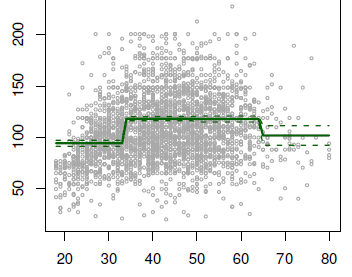# Statistics - (Interaction|Synergy) effect

In a multiple regression, is assumed that the effect on the target of increasing one unit of one predictor (is independent|has no influence) on the other predictor.

If this is not the case, sharing a value on two predictors, may have more effect than allocating the entire value to only one.

In marketing, this is known as a synergy effect, and in statistics it is referred to as an interaction effect.

The interaction effect is when the effect of one independent variable depends on the other.

The effect of one IV depends on the other IV (the simple effects of one IV change across the levels of the other IV)

This is exactly like a moderation effect in multiple regression.

This effect is called:

If the simple effects change as a function of the other IV, then you've got an interaction.

## Example

example of an interaction in a 2 X 3 factorial ANOVA:

• the effect of A is significant (but different in magnitude) at each level of B
• the effect of B is significant at one level of A but not at the other level of A
• the effect B is significant (but different in direction) at each level of A

example of no interaction in a 2 X 3 factorial ANOVA:

• the effect of A is significant (and the same magnitude) at each level of B

## Modelling interactions

### between quantitative variables

Introduction of a new variable: the (product|interaction) term. $X_{1i} . X_{2i}$

$$\begin{array}{ll} Y_i & = & B_0 + B_1 X_{1i} + B_2 X_{2i} + B_3 ( X_{1i} . X_{2i} ) + \epsilon_i \\ & = & B_0 + (B_1 + B_3 X_{2i}) X_{1i} + B_2 X_{2i} + \epsilon_i \\ & = & B_0 + B_1 X_{1i} + (B_2 + B_3 X_{1i}) X_{2i} + \epsilon_i \end{array}$$ In the second and third formula form, we got only two variables but we can see that one is dependant of the other.

Interpretation:

• for one unit increase in $X_2$ , there is $(B_1 + B_3 X_{2i})$ increase in $X_1$
• for one unit increase in $X_1$ , there is $(B_2 + B_3 X_{1i})$ increase in $X_2$

If the p-value for the interaction term is extremely low, it indicates that there is strong evidence that $H_A : B_ 3 \neq 0$ .

If R2 for the interaction model has augmented, it means that more variability has been explained by the interaction term.

### Hierarchy

Sometimes an interaction term has a very small p-value, but the associated main effects do not (ie $X_1 \text{and} X_2$ )

The hierarchy principle:

If we include an interaction in a model, we should also include the main effects, even if the p-values associated with their coefficients are not significant.

The rationale for this principle is that interactions are hard to interpret in a model without main effects (their meaning is changed).

Specifically, the interaction terms also contain main effects, if the model has no main effect terms.

### between qualitative and quantitative variables

Without an interaction term, the model takes the form:

$$\begin{array}{ll} Y_i & \approx & B_0 + B_1 X_i + \left\{ \begin{array}{ll} B_2 & \text{ if the ith person is a male} \\ 0 & \text{ if the ith person is a female} \end{array}\right. \\ & = &B_1 X_i + \left\{ \begin{array}{ll} B_0 + B_2 & \text{ if the ith person is a male} \\ B_0 & \text{ if the ith person is a female} \end{array}\right. \end{array}$$

By grouping the items (Second line formula), we can think of this as having a common slope but a different intercept depending on whether the person is a male or female.

By putting an interaction term, we're going to get both a different intercept and a different slope. The model takes the form:

If you're not a male, the term is 0, which means you get the baseline intercept and slope.

$$\begin{array}{ll} Y_i & \approx & B_0 + B_1 X_i + \left\{ \begin{array}{ll} B_2 + B_3 X_i & \text{ if the ith person is a male} \\ 0 & \text{ if the ith person is a female} \end{array}\right. \\ & = & \left\{ \begin{array}{ll} (B_0 + B_2) + (B_1 + B_3) X_i & \text{ if the ith person is a male} \\ B_0 + B_1 X_i & \text{ if the ith person is a female} \end{array}\right. \end{array}$$

Recommended PagesData Mining - Step Function (piecewise constants)

Step functions, are another way of fitting non-linearities. (especially popular in epidemiology and biostatistics) Continuous variable are cut into discrete sub-ranges and fit a constant model in...R - Interaction Analysis

interaction with R . An interaction term between a numeric x and z is just the product of x and z. lm processes the “” operator between variables andautomatically: add the interaction...Statistics - Analysis of variance (Anova)

Anova is just a special case of multiple regression. There're many forms of ANOVA. It's a very common procedure in basic statistics. Anova is more Appropriate when: there's true independent variable...Statistics - Effects (between predictor variable)Statistics - Factorial Anova

A factorial ANOVA is done when the independent variables are categorical. By adding a second independent variable, we are entering in factorial ANOVA. N Independent Variables (IVs). Variables that...Statistics - Generalized Linear Models (GLM) - Extensions of the Linear Model

The Generalized Linear Model is an extension of the linear model that allows for lots of different,non-linear models to be tested in the context of regression. GLM is the mathematical framework used in...Statistics - Simple Effect

The effect of one IV at a particular (level|value) of the other IV. If the simple effects change as a function of the other IV, then you've got an interaction.